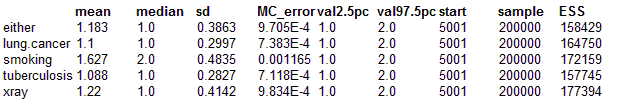#### Asia: expert system

Evidence propagation

Lauritzen and Spiegelhalter (1988) introduce a fictitious "expert system" representing the diagnosis of a patient presenting to a chest clinic, having just come back from a trip to Asia and showing dyspnoea (shortness-of-breath). The BUGS code is shown below and the conditional probabilities used are given in Lauritzen and Spiegelhalter (1988). Note the use of max to do the logical-or. The dcat distribution is used to sample values with domain (1,2) with probability distribution given by the relevant entries in the conditional probability
tables. model
{
smoking ~ dcat(p.smoking[1:2])
tuberculosis ~ dcat(p.tuberculosis[asia,1:2])
lung.cancer ~ dcat(p.lung.cancer[smoking,1:2])
bronchitis ~ dcat(p.bronchitis[smoking,1:2])
either <- max(tuberculosis,lung.cancer)
xray ~ dcat(p.xray[either,1:2])
dyspnoea ~ dcat(p.dyspnoea[either,bronchitis,1:2])
}

##### Data
``` list(asia = 2, dyspnoea = 2,      p.tuberculosis = structure(.Data = c(0.99,0.01,0.95,0.05), .Dim = c(2,2)),      p.bronchitis = structure(.Data = c(0.70,0.30,0.40,0.60), .Dim = c(2,2)),      p.smoking = c(0.50,0.50),      p.lung.cancer = structure(.Data = c(0.99,0.01,0.90,0.10), .Dim = c(2,2)),      p.xray = structure(.Data = c(0.95,0.05,0.02,0.98), .Dim = c(2,2)),      p.dyspnoea = structure(.Data = c(0.9,0.1,      0.2,0.8,      0.3,0.7,      0.1,0.9), .Dim = c(2,2,2))) ```
##### Inits for chain 1
``` list(smoking = 1, tuberculosis = 1, lung.cancer = 1, bronchitis = 1, xray = 1) ```

##### Inits for chain 2
``` list(smoking = 2, tuberculosis = 2, lung.cancer = 2, bronchitis = 2, xray = 2) ```
Results